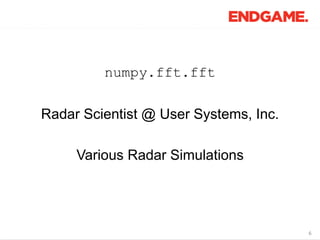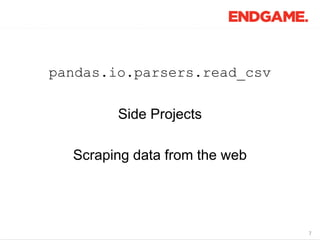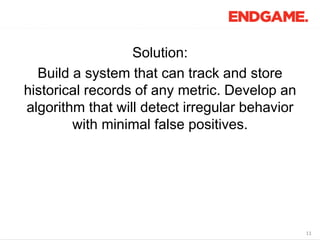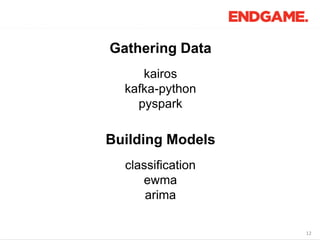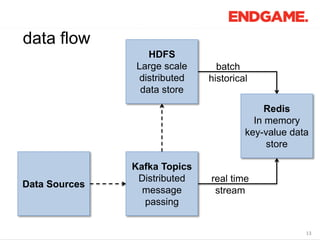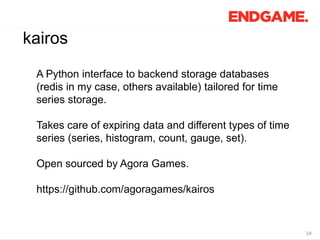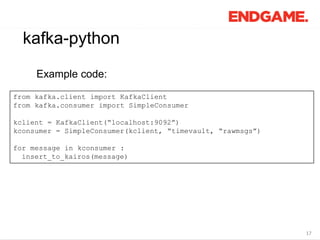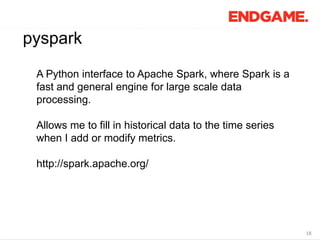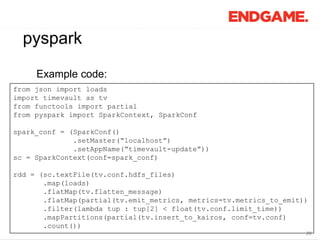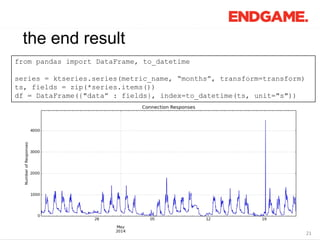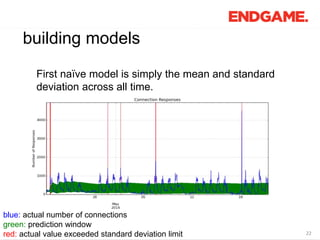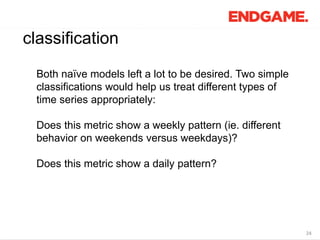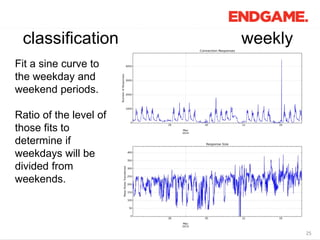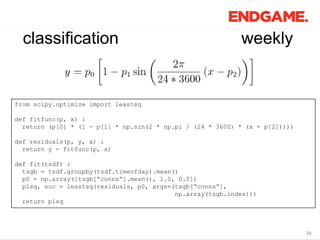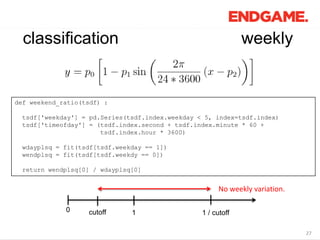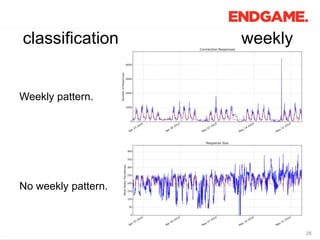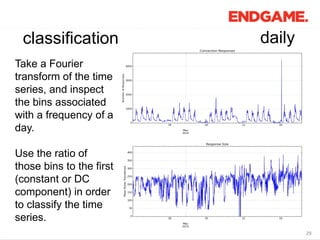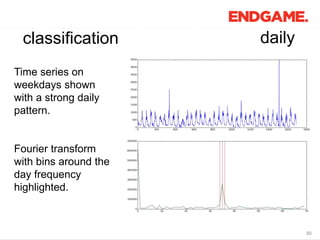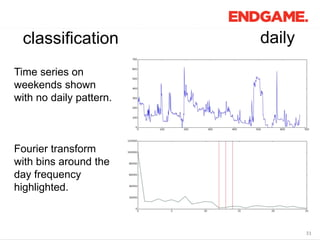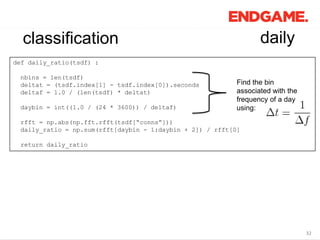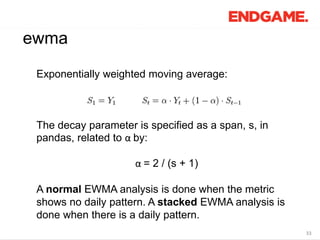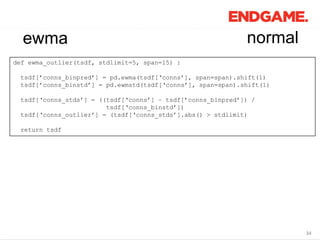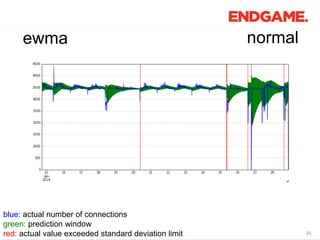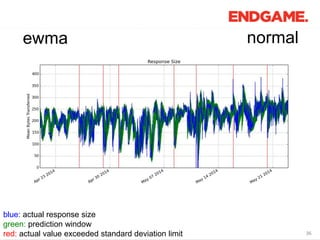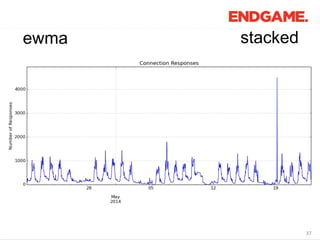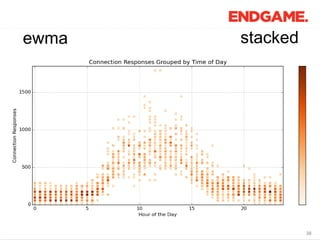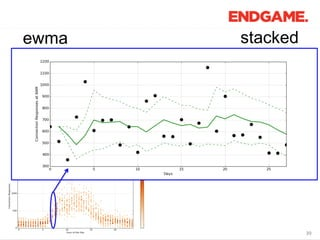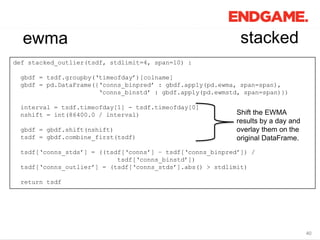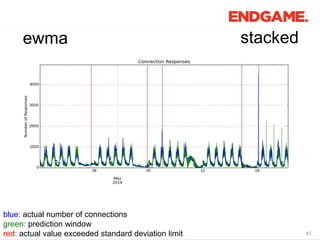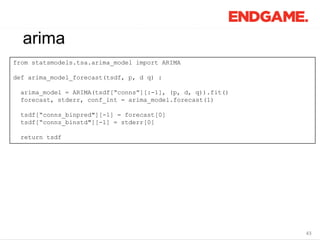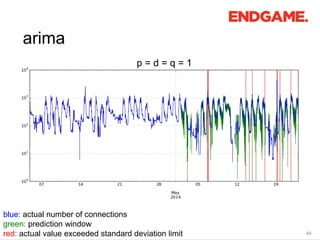1 of 46

### Time Series Analysis for Network Secruity

1. 1 Endgame Proprietary
2. 2 Time Series Analysis for Network Security Phil Roth Data Scientist @ Endgame mrphilroth.com
3. 33 First, an introduction. My history of Python scientific computing, in function calls:
4. 44 os.path.walk Physics Undergraduate @ PSU AMANDA Neutrino Telescope
5. 55 pylab.plot Physics Graduate Student @ UMD IceCube Neutrino Telescope
6. 66 numpy.fft.fft Radar Scientist @ User Systems, Inc. Various Radar Simulations
7. 77 pandas.io.parsers.read_csv Side Projects Scraping data from the web
8. 88 sklearn.linear_model.LogisticRegression Side Projects Machine learning competitions
9. 99 (the rest of this talk…) Data Scientist @ Endgame Time Series Anomaly Detection
10. 1010 Problem: Highlight when recorded metrics deviate from normal patterns. for example: a high number of connections might be an indication of a brute force attack for example: a large volume of outgoing data might be an indication of an exfiltration event
11. 1111 Solution: Build a system that can track and store historical records of any metric. Develop an algorithm that will detect irregular behavior with minimal false positives.
12. 1212 Gathering Data kairos kafka-python pyspark Building Models classification ewma arima
13. 1313 real time stream batch historical Redis In memory key-value data store HDFS Large scale distributed data store Kafka Topics Distributed message passing Data Sources data flow
14. 1414 kairos A Python interface to backend storage databases (redis in my case, others available) tailored for time series storage. Takes care of expiring data and different types of time series (series, histogram, count, gauge, set). Open sourced by Agora Games. https://github.com/agoragames/kairos
15. 1515 kairos Example code: from redis import Redis from kairos import Timeseries intervals = {"days" : {"step" : 60, "steps" : 2880}, "months" : {"step" : 1800, "steps" : 4032}} rclient = Redis(“localhost”, 6379) ktseries = Timeseries(rclient, type="histogram”, intervals=intervals) ktseries.insert(metric_name, metric_value, timestamp)
16. 1616 kafka-python A Python interface to Apache Kafka, where Kafka is publish-subscribe messaging rethought as a distributed commit log. Allows me to subscribe to the events as they come in real time. https://github.com/mumrah/kafka-python
17. 1717 kafka-python from kafka.client import KafkaClient from kafka.consumer import SimpleConsumer kclient = KafkaClient(“localhost:9092”) kconsumer = SimpleConsumer(kclient, “timevault, “rawmsgs”) for message in kconsumer : insert_to_kairos(message) Example code:
18. 1818 pyspark A Python interface to Apache Spark, where Spark is a fast and general engine for large scale data processing. Allows me to fill in historical data to the time series when I add or modify metrics. http://spark.apache.org/
19. 1919 pyspark from pyspark import SparkContext, SparkConf spark_conf = (SparkConf() .setMaster(“localhost”) .setAppName(“timevault-update”)) sc = SparkContext(conf=spark_conf) rdd = (sc.textFile(hdfs_files) .map(insert_to_kairos) .count()) Example code:
20. 2020 pyspark from json import loads import timevault as tv from functools import partial from pyspark import SparkContext, SparkConf spark_conf = (SparkConf() .setMaster(“localhost”) .setAppName(“timevault-update”)) sc = SparkContext(conf=spark_conf) rdd = (sc.textFile(tv.conf.hdfs_files) .map(loads) .flatMap(tv.flatten_message) .flatMap(partial(tv.emit_metrics, metrics=tv.metrics_to_emit)) .filter(lambda tup : tup < float(tv.conf.limit_time)) .mapPartitions(partial(tv.insert_to_kairos, conf=tv.conf) .count()) Example code:
21. 2121 the end result from pandas import DataFrame, to_datetime series = ktseries.series(metric_name, “months”, transform=transform) ts, fields = zip(*series.items()) df = DataFrame({"data” : fields}, index=to_datetime(ts, unit="s"))
22. 2222 building models First naïve model is simply the mean and standard deviation across all time. blue: actual number of connections green: prediction window red: actual value exceeded standard deviation limit
23. 2323 building models Second slightly less naïve model is fitting a sine curve to the whole series. blue: actual number of connections green: prediction window red: actual value exceeded standard deviation limit
24. 2424 classification Both naïve models left a lot to be desired. Two simple classifications would help us treat different types of time series appropriately: Does this metric show a weekly pattern (ie. different behavior on weekends versus weekdays)? Does this metric show a daily pattern?
25. 2525 classification Fit a sine curve to the weekday and weekend periods. Ratio of the level of those fits to determine if weekdays will be divided from weekends. weekly
26. 2626 classification weekly from scipy.optimize import leastsq def fitfunc(p, x) : return (p * (1 - p * np.sin(2 * np.pi / (24 * 3600) * (x + p)))) def residuals(p, y, x) : return y - fitfunc(p, x) def fit(tsdf) : tsgb = tsdf.groupby(tsdf.timeofday).mean() p0 = np.array([tsgb[“conns”].mean(), 1.0, 0.0]) plsq, suc = leastsq(residuals, p0, args=(tsgb[“conns”], np.array(tsgb.index))) return plsq
27. 2727 classification weekly def weekend_ratio(tsdf) : tsdf['weekday'] = pd.Series(tsdf.index.weekday < 5, index=tsdf.index) tsdf['timeofday'] = (tsdf.index.second + tsdf.index.minute * 60 + tsdf.index.hour * 3600) wdayplsq = fit(tsdf[tsdf.weekday == 1]) wendplsq = fit(tsdf[tsdf.weekdy == 0]) return wendplsq / wdayplsq 0 1cutoff 1 / cutoff No weekly variation.
28. 2828 classification Weekly pattern. No weekly pattern. weekly
29. 2929 classification Take a Fourier transform of the time series, and inspect the bins associated with a frequency of a day. Use the ratio of those bins to the first (constant or DC component) in order to classify the time series. daily
30. 3030 classification Time series on weekdays shown with a strong daily pattern. Fourier transform with bins around the day frequency highlighted. daily
31. 3131 classification Time series on weekends shown with no daily pattern. Fourier transform with bins around the day frequency highlighted. daily
32. 3232 classification def daily_ratio(tsdf) : nbins = len(tsdf) deltat = (tsdf.index - tsdf.index).seconds deltaf = 1.0 / (len(tsdf) * deltat) daybin = int((1.0 / (24 * 3600)) / deltaf) rfft = np.abs(np.fft.rfft(tsdf[“conns”])) daily_ratio = np.sum(rfft[daybin - 1:daybin + 2]) / rfft return daily_ratio daily Find the bin associated with the frequency of a day using:
33. 3333 ewma Exponentially weighted moving average: The decay parameter is specified as a span, s, in pandas, related to α by: α = 2 / (s + 1) A normal EWMA analysis is done when the metric shows no daily pattern. A stacked EWMA analysis is done when there is a daily pattern.
34. 3434 ewma def ewma_outlier(tsdf, stdlimit=5, span=15) : tsdf[’conns_binpred’] = pd.ewma(tsdf[‘conns’], span=span).shift(1) tsdf[’conns_binstd’] = pd.ewmstd(tsdf[‘conns’], span=span).shift(1) tsdf[‘conns_stds’] = ((tsdf[‘conns’] – tsdf[’conns_binpred’]) / tsdf[‘conns_binstd’]) tsdf[‘conns_outlier’] = (tsdf[‘conns_stds’].abs() > stdlimit) return tsdf normal
35. 3535 ewma normal blue: actual number of connections green: prediction window red: actual value exceeded standard deviation limit
36. 3636 ewma blue: actual response size green: prediction window red: actual value exceeded standard deviation limit normal
37. 3737 ewma stacked
38. 3838 ewma stacked
39. 3939 ewma stacked
40. 4040 ewma def stacked_outlier(tsdf, stdlimit=4, span=10) : gbdf = tsdf.groupby(‘timeofday’)[colname] gbdf = pd.DataFrame({‘conns_binpred’ : gbdf.apply(pd.ewma, span=span), ‘conns_binstd’ : gbdf.apply(pd.ewmstd, span=span)}) interval = tsdf.timeofday - tsdf.timeofday nshift = int(86400.0 / interval) gbdf = gbdf.shift(nshift) tsdf = gbdf.combine_first(tsdf) tsdf[‘conns_stds’] = ((tsdf[‘conns’] – tsdf[‘conns_binpred’]) / tsdf[‘conns_binstd’]) tsdf[‘conns_outlier’] = (tsdf[‘conns_stds’].abs() > stdlimit) return tsdf stacked Shift the EWMA results by a day and overlay them on the original DataFrame.
41. 4141 ewma blue: actual number of connections green: prediction window red: actual value exceeded standard deviation limit stacked
42. 4242 arima I am currently investigating using ARIMA (autoregressive integrated moving average) models to make better predictions. I’m not convinced that this level of detail is necessary for the analysis I’m doing, but I wanted to highlight another cool scientific computing library that’s available.
43. 4343 arima from statsmodels.tsa.arima_model import ARIMA def arima_model_forecast(tsdf, p, d q) : arima_model = ARIMA(tsdf[“conns”][:-1], (p, d, q)).fit() forecast, stderr, conf_int = arima_model.forecast(1) tsdf[“conns_binpred"][-1] = forecast tsdf[“conns_binstd"][-1] = stderr return tsdf
44. 4444 arima blue: actual number of connections green: prediction window red: actual value exceeded standard deviation limit p = d = q = 1
45. 4545 takeaways Python provides simple and usable interfaces to most data handling projects. Combined, these interfaces can create a full data analysis pipeline from collection to analysis.